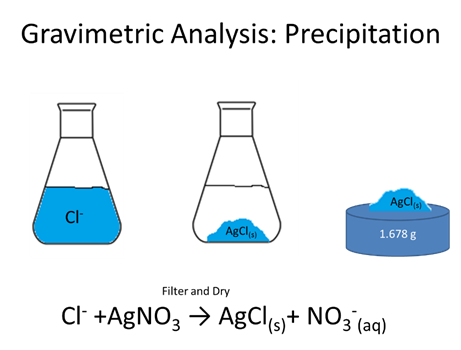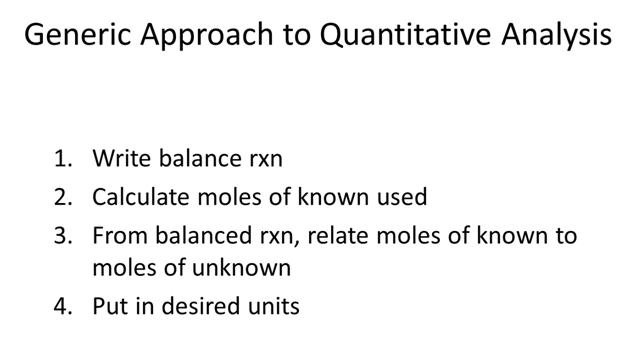# Gravimetric Analysis (Fry-Petit)

Reading: Chapter 7 (sections 1, 3), Exploring Chemical Analysis 5th ed., D. Harris

Homework: Chapter 7:2-7, 9, 10, 14-16, 18, 21.a• How many moles of green K(g) (FW=39.0983 g/mol) are evolved?STOPHow many moles Cl- were in the initial solution (AgCl, FW=143.321 g/mol) are evolved?

STOP

• As a group make a list of what you would want in a precipitate

STOP• In her Ph.D. research (Radioactive Substances, 1903), Marie Curie measured the atomic mass of the element radium, which she discovered. She knew that radium is in the same family as barium, so the formula of radium chloride is RaCl2. When 0.09192 g of pure RaCl2 was dissolved and treated with excess AgNO3, 0.08890 g of AgCl precipitated. How many moles of Cl- were in the RaCl2?

STOP

• A mixture containing only Al2O3 (FW: 101.96) and Fe2O3 (FW: 159.69) weights 2.019 g. When heated under a stream of H2(g), the Al2O3 is unchanged, but the Fe2O3 is converted to metallic Fe plus H2O(g). If the residue weights 1.774g, what is the weight percent of Fe2O3 in the original mixture?

STOP

• A 1.475g sample containing NH4Cl (FW 53.492), K2CO3 (FW 138.21), and inert ingredients was dissolved to give 0.100 L of solution. A 25.0 mL aliquot was acidified and treated with excess sodium tetrapheylborate, Na+B(C6H5)4-, to precipitate K+ and NH4+ ions completely:

$\ce{B(C6H5)4- +K+ → (C6H5)4BK_{(s)}}\: \textrm{(FW 358.33)}\nonumber$

$\ce{B(C6H5)4- + NH4+ → (C6H5)4B NH4_{(s)}}\: \textrm{(FW 337.27)}\nonumber$

The resulting precipitate amounted to 0.617g. A fresh 50.0 mL aliquot of the original solution was made alkaline and heated to drive off all the NH3:

$\ce{NH+4 + OH- → NH3_{(g)} + H2O}\nonumber$

It was then acidified and treated with sodium tetraphenyl borate to give 0.554 g of precipitate. Find the weight percent of NH4Cl and K2CO3 in the original solid.

STOP